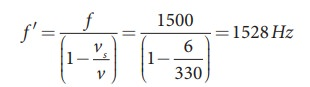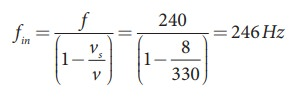Home | | Physics 11th std | Solved Example Problems for Doppler Effect

# Solved Example Problems for Doppler Effect

Physics : Waves - Solved Example Problems for Doppler Effect

### EXAMPLE 11.30

A sound of frequency 1500 Hz is emitted by a source which moves away from an observer and moves towards a cliff at a speed of 6 ms-1.

(a) Calculate the frequency of the sound which is coming directly from the source.

(b) Compute the frequency of sound heard by the observer reflected off the cliff. Assume the speed of sound in air is 330 m s-1

### Solution

(a) Source is moving away and observer is stationary, therefore, the frequency of sound heard directly from source is(b) Sound is reflected from the cliff and reaches observer, therefore,### EXAMPLE 11.31

An observer observes two moving trains, one reaching the station and other leaving the station with equal speeds of 8 m s−1. If each train sounds its whistles with frequency 240 Hz, then calculate the number of beats heard by the observer.

### Solution:

Observer is stationary

(i) Source (train) is moving towards an observer:

Apparent frequency due to train arriving station is(ii) Source (train) is moving away form an observer:

Apparent frequency due to train leaving station isSo the number of beats = | fin -fout| = (246-234) = 12

Tags : Waves | Physics , 11th Physics : UNIT 11 : Waves
Study Material, Lecturing Notes, Assignment, Reference, Wiki description explanation, brief detail
11th Physics : UNIT 11 : Waves : Solved Example Problems for Doppler Effect | Waves | Physics